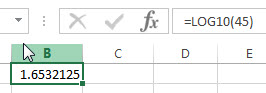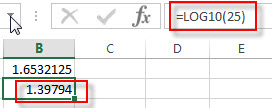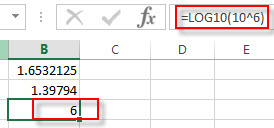# Excel LOG10 Function

This post will guide you how to use Excel LOG10 function with syntax and examples in Microsoft excel.

### Description

The Excel LOG10 function returns the base-10 logarithm of a given number.

The LOG10 function is a build-in function in Microsoft Excel and it is categorized as a Math and Trigonometry Function.

The LOG10 function is available in Excel 2016, Excel 2013, Excel 2010, Excel 2007, Excel 2003, Excel XP, Excel 2000, Excel 2011 for Mac.

### Syntax

The syntax of the LOG10 function is as below:

`= LOG10 (number)`

Where the LOG10 function arguments are:

• number – This is a required argument. The number that you want the logarithm. And the number must be a positive real number.

Note:

• If the number argument is negative or zero, the LOG10 function will return #NUM! Error.
• If the number argument is non-numeric, the LOG10 function will return #VALUE! Error.

### Excel LOG10 Function Examples

The below examples will show you how to use Excel LOG10 Function to calculate the base 10 logarithm of a given number.

1# to get the base 10 logarithm of 45, enter the following formula in Cell B1.

`=LOG10(45)`2# to get the base 10 logarithm of 25, enter the following formula in Cell B2.

`=LOG10(25)`3# to get the base 10 logarithm of 10 ^6, enter the following formula in Cell B2.

`=LOG10(10^6)`### Related Functions

• Excel LOG function
The Excel LOG function returns the logarithm of a given number based on a supplied base. And the default base argument is 10.The syntax of the LOG function is as below:= LOG (number,[base])…
• Excel LN function
The Excel LN function returns the natural logarithm of a given number.  And the natural logarithms are based on the constant e (the value is equal to 2.718281828…). The syntax of the LN function is as below:= LN (number)…